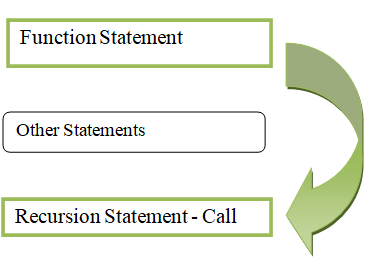# Understanding Recursive Functions with Python

• Last Updated : 15 Jul, 2021

Recursion is characterized as the process of describing something in terms of itself; in other words, it is the process of naming the function by itself. Recursion is the mechanism of a function calling itself directly or implicitly, and the resulting function is known as a Recursive function.

• Code reusability
• Easily understandable
• Time complexity sometimes low
• Less number of code

• Causes overflow if condition exceeds
• More memory
• It is difficult to understand
• It is difficult to debug
• Terminating conditions in Recursion is a must

Syntax:

Attention geek! Strengthen your foundations with the Python Programming Foundation Course and learn the basics.

To begin with, your interview preparations Enhance your Data Structures concepts with the Python DS Course. And to begin with your Machine Learning Journey, join the Machine Learning - Basic Level Course

def function_name():

……………..

|(recursive call)

………………….

function_name()Recursion calls the function which is already called and will call many times till the condition will become false. After that, it will return the value

### Applications:

• Tower of Hanoi
• Stack implementation
• Fibonacci implementation
• Round Robin problem in Operating System.

Apart from the above applications below are some examples that depict how to use recursive functions in a program.

Example 1:

Python program to print Fibonacci series up to given terms.

Fibonacci:

Number=sum of two previous numbers

0,1

0+1=1

0+1=2

2+1=3

3+2=5

5+3=8

So the Fibonacci numbers are 0,1,1,2,3,5,8…….

In this program, the values are defined in till_range variables and then we are passing that variable into an argument, then calling the recursive function.

`recursive_function(a-1) + recursive_function(a-2)`

Implementation:

## Python3

 `# Recursive function``def` `recursive_function(a):``  ` `    ``# Check number``    ``if` `a <``=` `1``:``        ``return` `a``    ``else``:``        ` `        ``# Get next term``        ``return``(recursive_function(a``-``1``) ``+` `recursive_function(a``-``2``))` `    ` `# Display first fibonacci number``print``(``"\nFibonacci series upto 1 number:"``)``for` `i ``in` `range``(``1``):``    ``print``(recursive_function(i),``          ``end``=``" "``)   ``    ` `# Display first 5 fibonacci numbers``print``(``"\nFibonacci series upto 5 numbers:"``)``for` `i ``in` `range``(``5``):``    ``print``(recursive_function(i),``          ``end``=``" "``)``    ` `# Display first 10 fibonacci numbers``print``(``"\nFibonacci series upto 10 numbers:"``)``for` `i ``in` `range``(``10``):``    ``print``(recursive_function(i),``          ``end``=``" "``)   `

Output:

```Fibonacci series upto 1 number:
0
Fibonacci series upto 5 numbers:
0 1 1 2 3
Fibonacci series upto 10 numbers:
0 1 1 2 3 5 8 13 21 34 ```

Example 2:

Python program to find factorial of a number.

Factorial

Number=Number*(Number-1)*(Number-2)…*(Number-(Number+1))

Factorial of 3= 3*2*1

Factorial of 5= 5*4*3*2*1

Factorial of 7 = 7*6*5*4*3*2*1

In this python program, we are going to return the factorial of an integer. We are passing the variable n value to an argument, then we are calling the function.

`a* recursion_factorial(a-1)`

Implementation:

## Python3

 `# recursive function``def` `recursion_factorial(a):``  ` `    ``# check number``    ``if` `a ``=``=` `1``:``        ``return` `1``    ``else``:``        ` `        ``# multiply with next number``        ``return` `(a ``*` `recursion_factorial(a``-``1``))`  `# factorial of 7``n ``=` `7``print``(``"Factorial of"``, n, ``"is"``, recursion_factorial(n))` `# factorial of 2``n ``=` `2``print``(``"Factorial of"``, n, ``"is"``, recursion_factorial(n))` `# factorial of 4``n ``=` `4``print``(``"Factorial of"``, n, ``"is"``, recursion_factorial(n))` `# factorial of 9``n ``=` `9``print``(``"Factorial of"``, n, ``"is"``, recursion_factorial(n))` `# factorial of 10``n ``=` `10``print``(``"Factorial of"``, n, ``"is"``, recursion_factorial(n))`

Output:

```Factorial of 7 is 5040
Factorial of 2 is 2
Factorial of 4 is 24
Factorial of 9 is 362880
Factorial of 10 is 3628800```

My Personal Notes arrow_drop_up